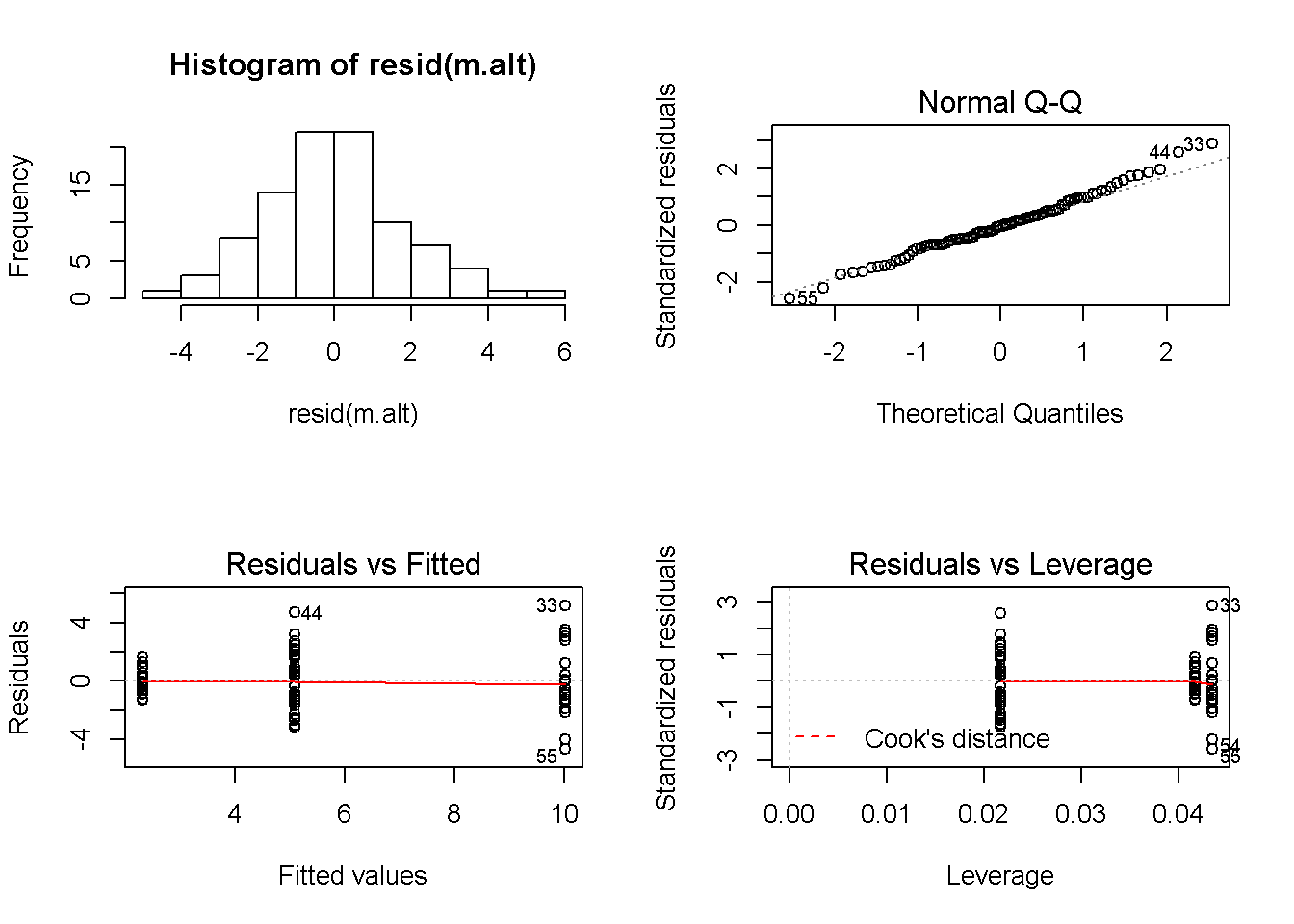Lion data set as 1-way ANOVA

#The following sets up the data fro the analysis
#Set working directory

setwd("C:/Users/lisanjie2/Desktop/TEACHING/1_STATS_CalU/1_STAT_CalU_2016_by_NLB/Lecture/Unit3_regression/last_week")

pigment.fivenum <- fivenum(dat$portion.black) plot(age.years ~ portion.black, data = dat, main = "Lion pigment data split into 3 groups", ylim = c(0,19))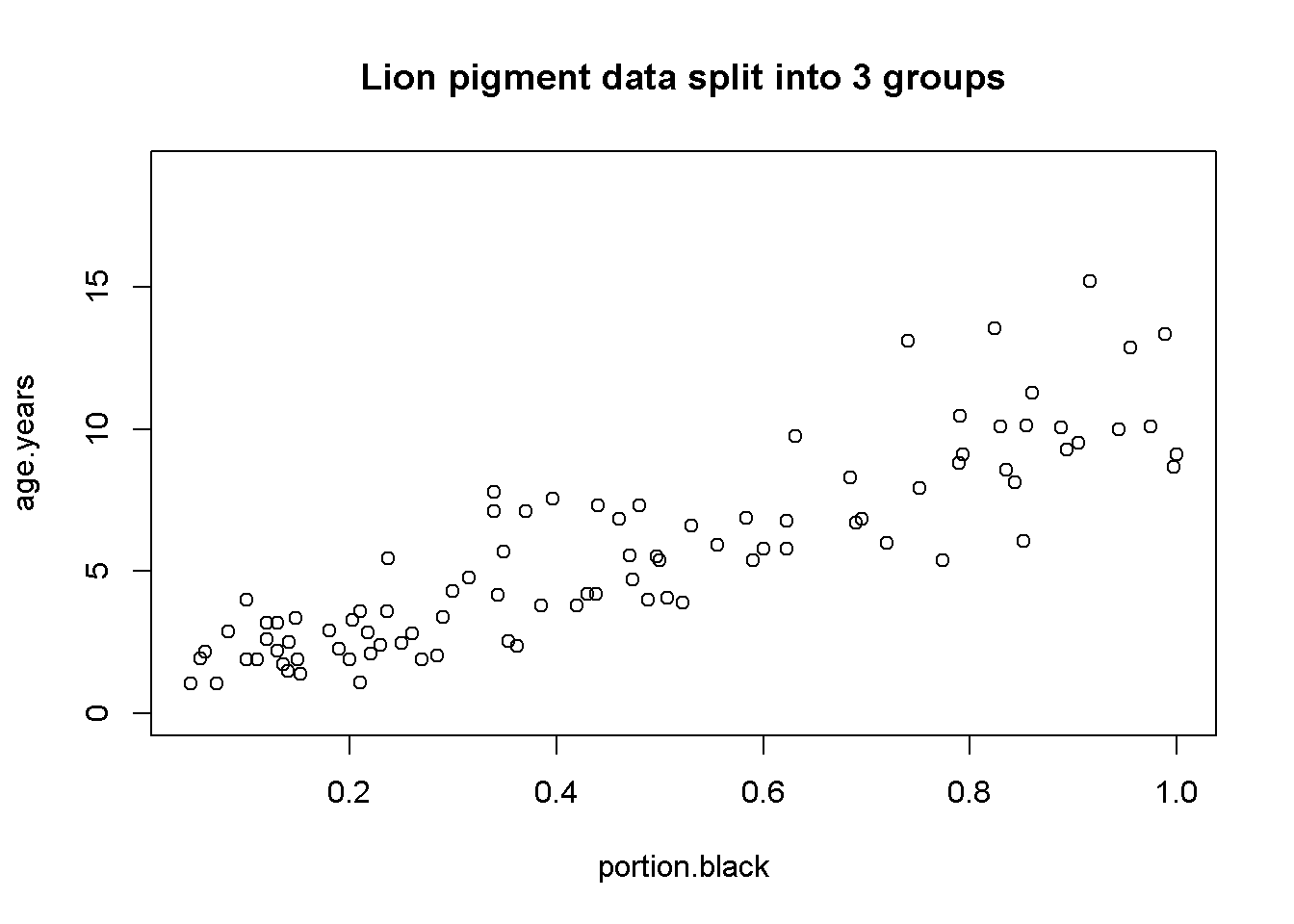Split lion data by groups pigment.fivenum <- fivenum(dat$portion.black)
plot(age.years ~ portion.black, data = dat,
main = "Lion pigment data split into 3 groups",
ylim = c(0,19))
abline(v = pigment.fivenum, col = 2, lwd = 3)
abline(v = pigment.fivenum, col = 2, lwd = 3)
text(x = 0.125, y = 17, "Low\npigment", cex =1.1)
text(x = 0.5, y = 17, "Mod.\npigment", cex = 1.1)
text(x = 0.895, y = 17, "High\npigment", cex = 1.1)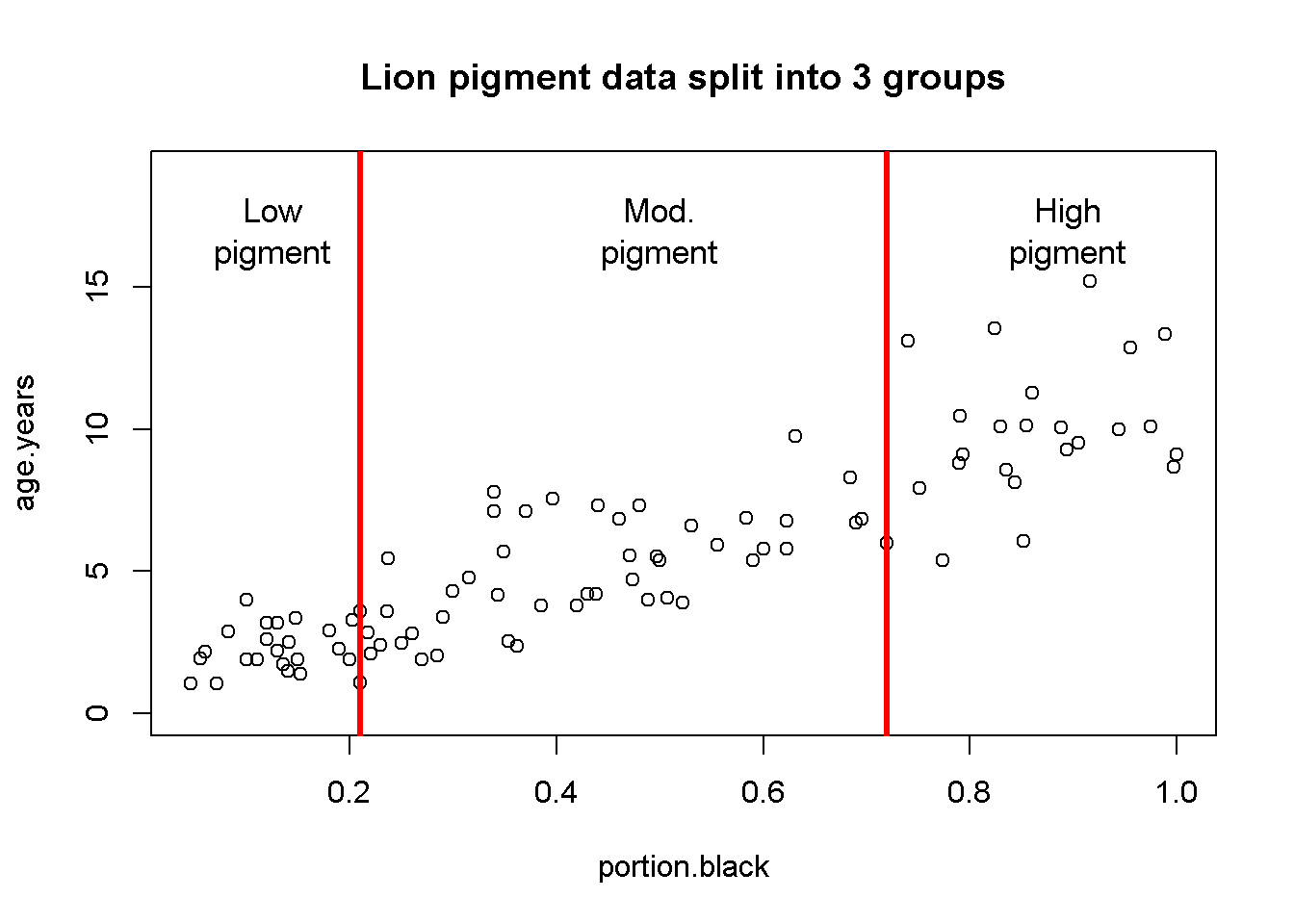Create 3 pigment groups

pigment.fivenum <- fivenum(dat$portion.black) dat$pigment.groups.3 <- "mod.pigment"

dat$pigment.groups.3[which(dat$portion.black <= pigment.fivenum[])] <- "low.pigment"

dat$pigment.groups.3[which(dat$portion.black > pigment.fivenum[])] <- "high.pigment"

dat$pigment.groups.3 <- factor(dat$pigment.groups.3)
summary(dat)
##    age.years      portion.black         population     sex
##  Min.   : 1.056   Min.   :0.04609   Ngorogoro:10   female:61
##  1st Qu.: 2.800   1st Qu.:0.21000   Serengeti:83   male  :32
##  Median : 5.378   Median :0.43000
##  Mean   : 5.589   Mean   :0.46446
##  3rd Qu.: 7.800   3rd Qu.:0.72000
##  Max.   :15.178   Max.   :1.00000
##      pigment.groups.3
##  high.pigment:23
##  low.pigment :24
##  mod.pigment :46
##
##
##
dat$pigment.groups.3 <- factor(dat$pigment.groups.3,
levels =c("low.pigment",
"mod.pigment",
"high.pigment"))

table(dat$pigment.groups.3, dat$population)
##
##                Ngorogoro Serengeti
##   low.pigment          1        23
##   mod.pigment          7        39
##   high.pigment         2        21
table(dat$pigment.groups.3, dat$sex)
##
##                female male
##   low.pigment      11   13
##   mod.pigment      29   17
##   high.pigment     21    2

NOTE: Splitting up a continuous variable like this into a categorical variable and doing an ANOVA is generally a BAD idea. Regression is the proper way to analyze the raw data. I am making these groups for illustration purposes only!

Plot raw data by group

par(mfrow = c(1,1))
plot(age.years ~ pigment.groups.3,data = dat,
xlab = "Pigmentation group",
ylab = "Known Lion Age")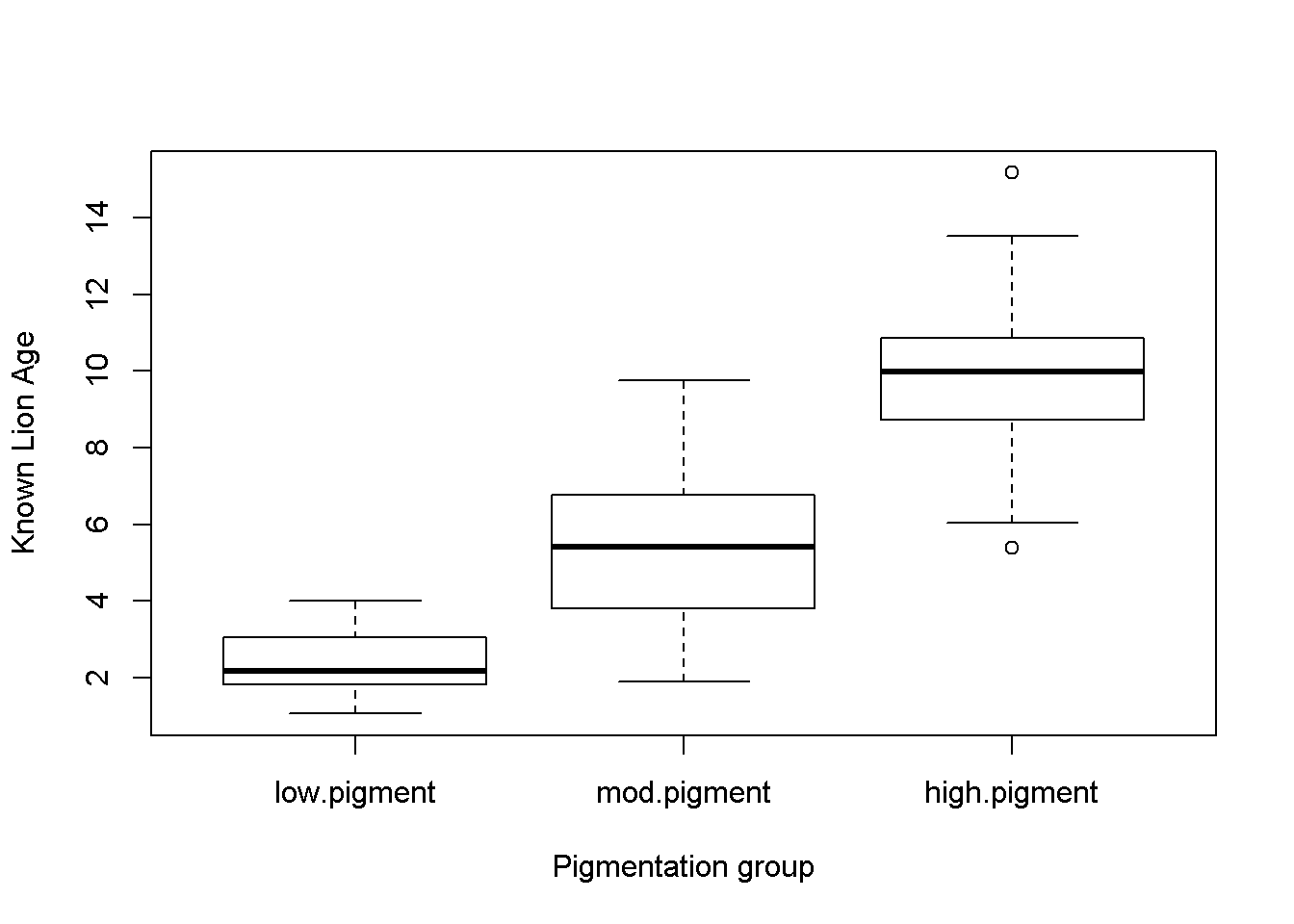Figure 1: Box plots of lion ages in three pigmentation groups from the Serengeti and Ngorogoro Crater, Tanzania, east Africa.

Plot means

library(sciplot)

ci.fun. <- function(x) c(mean(x)-2*se(x), mean(x)+3*se(x))
lineplot.CI(x.factor = pigment.groups.3,
response = age.years,
data = dat,
ci.fun = ci.fun.
)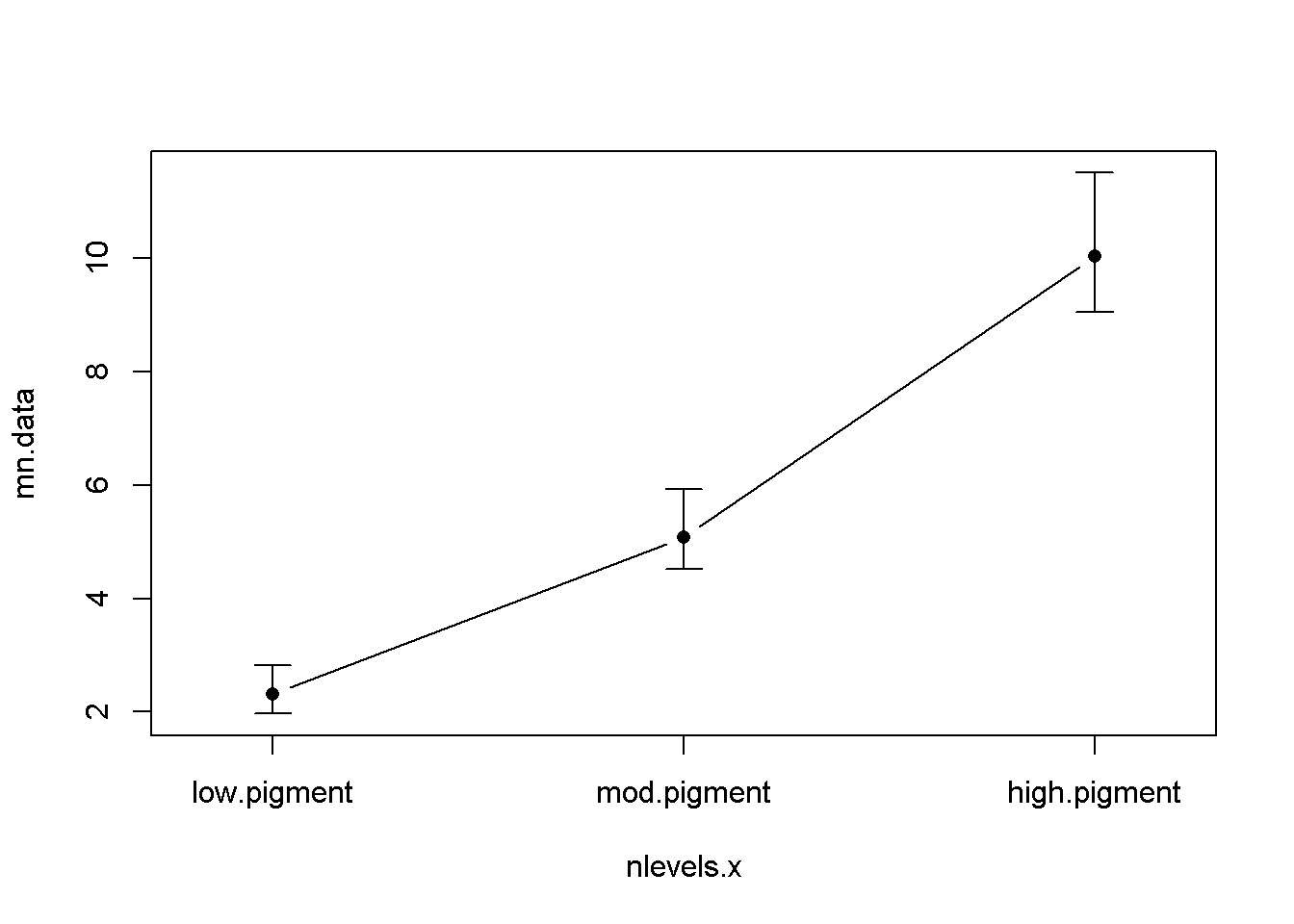Figure 2: Mean age of lions in three pigmentation groups from the Serengeti and Ngorogoro crater, Tanzania, east Africa. Error bars are approximate 95% confidence intervals.

1-way ANOVA

(again, don’t do an analysis like this - use the original data and do an ANOVA!)

Null model: age.years ~ 1

Age does not vary with pigmentation

m.null <- lm(age.years ~ 1 ,data = dat)

Alternative model: age.years ~ pigment.groups.3

Age does vary with pigmentation

m.alt <- lm(age.years ~ pigment.groups.3,data = dat)

Omnibus ANOVA F-test

anova(m.null, m.alt)
## Analysis of Variance Table
##
## Model 1: age.years ~ 1
## Model 2: age.years ~ pigment.groups.3
##   Res.Df     RSS Df Sum of Sq      F    Pr(>F)
## 1     92 1025.42
## 2     90  304.24  2    721.18 106.67 < 2.2e-16 ***
## ---
## Signif. codes:  0 '***' 0.001 '**' 0.01 '*' 0.05 '.' 0.1 ' ' 1

Writing up result of Omnibus F-test

Summarize reulst of ANOVA in a single sentence: “There was a significant difference in the mean ages of the three different pigmentation groups (F = 106.67, p < 0.00001, DF = 92,90)”

Comparisons using Tukey-HSD

Refit m.alt w/ aov()

dat$pigment.groups.3.rename <- gsub("pigment","",dat$pigment.groups.3)

m.alt.aov <- aov(age.years ~ pigment.groups.3.rename,data = dat)

Pairwise comparison w/TukeyHSD()

Run TukeyHSD()

my.tukey <- TukeyHSD(m.alt.aov)

Look at Tukey output

This lists the effect sizes (differences), their p-values and confidence intervals.

my.tukey
##   Tukey multiple comparisons of means
##     95% family-wise confidence level
##
## Fit: aov(formula = age.years ~ pigment.groups.3.rename, data = dat)
##
## $pigment.groups.3.rename ## diff lwr upr p adj ## low.-high. -7.707850 -8.986380 -6.429321 0e+00 ## mod.-high. -4.939613 -6.058570 -3.820657 0e+00 ## mod.-low. 2.768237 1.664931 3.871542 1e-07 Plot Tukey HSD Plot the effect sizes calculated by TurkeyHSD source("plot_tukey_HSD_code.R") plotTukeysHSD(my.tukey,x.axis.label = "") abline(h =0, col =2)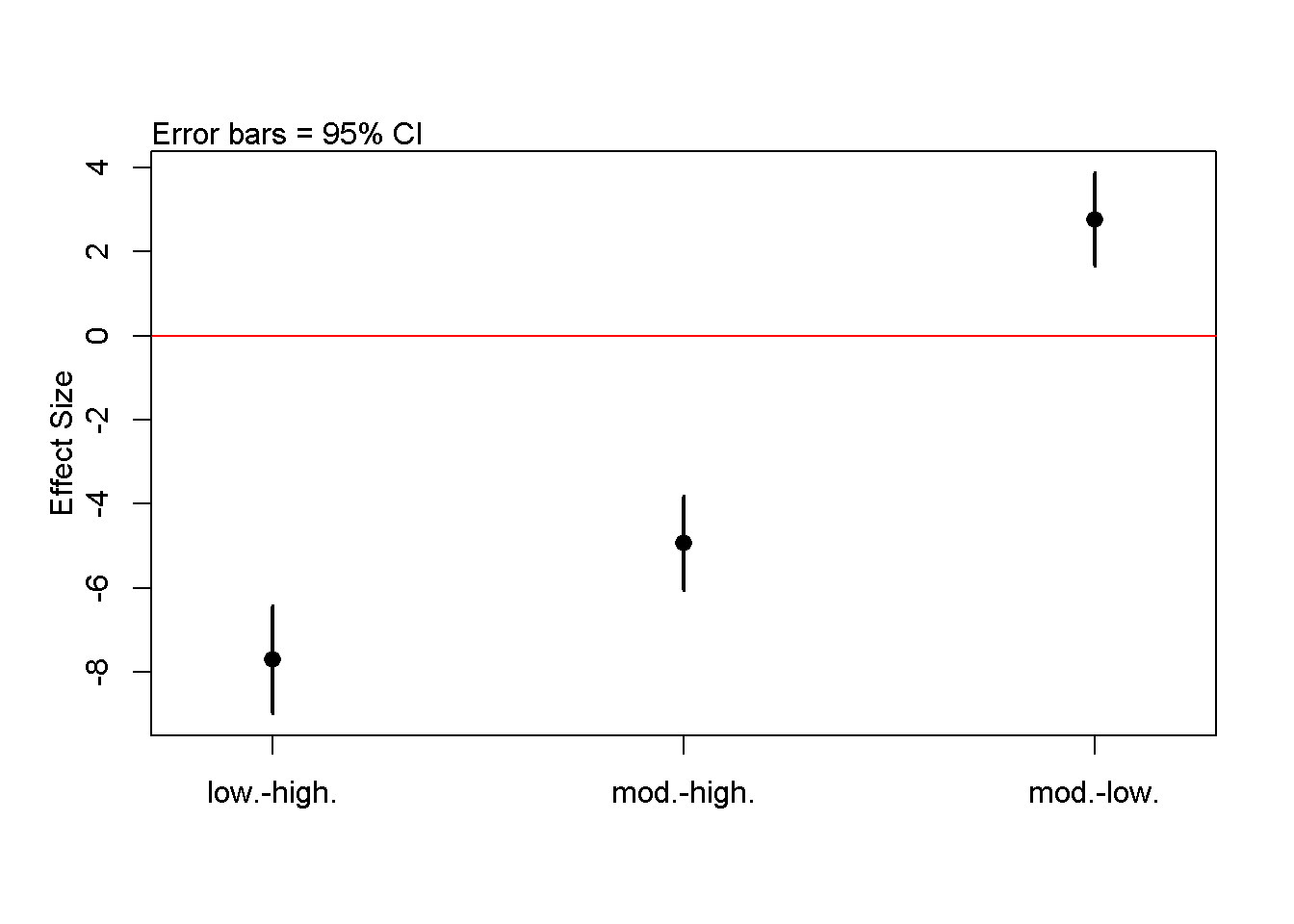Plot 3: Difference between mean lion ages (effect sizes) for three pigmentation groups calculated using Tukey’s HSD. Compare Means of raw data to plot of Tukey par(mfrow = c(1,2)) lineplot.CI(x.factor = pigment.groups.3, response = age.years, data = dat, ci.fun = ci.fun., main = "means of raw data") plotTukeysHSD(my.tukey,x.axis.label = "") abline(h = 0, col =2)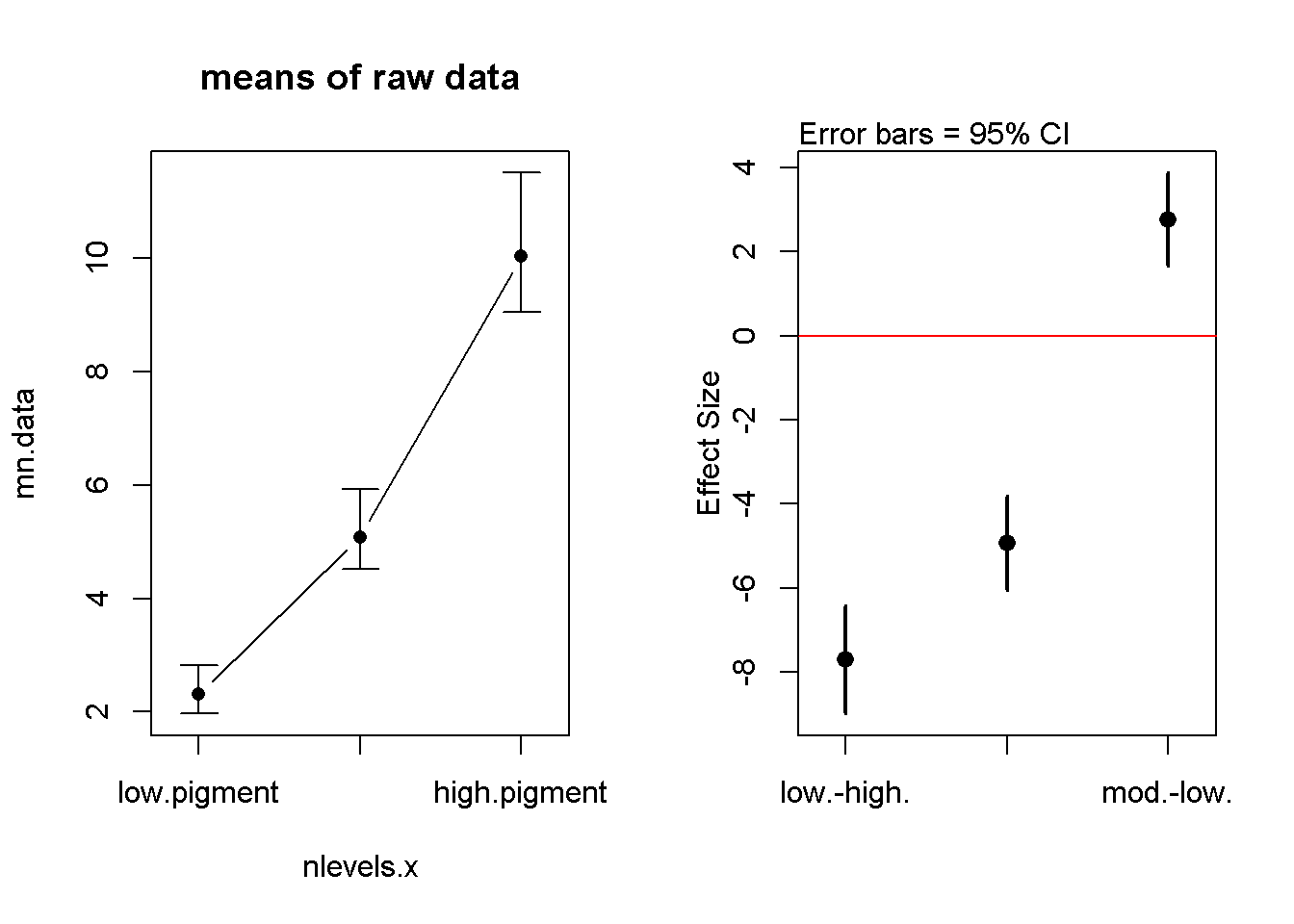## WRite results from Tukey my.tukey ## Tukey multiple comparisons of means ## 95% family-wise confidence level ## ## Fit: aov(formula = age.years ~ pigment.groups.3.rename, data = dat) ## ##$pigment.groups.3.rename
##                 diff       lwr       upr p adj
## low.-high. -7.707850 -8.986380 -6.429321 0e+00
## mod.-high. -4.939613 -6.058570 -3.820657 0e+00
## mod.-low.   2.768237  1.664931  3.871542 1e-07

The results could be written out like this: “Tukey’s HSD indicated that all means were different from each other. The difference between the low and moderate pigment groups was 2.7 years (p < 0.0001), the difference between the moderate and high groups was 4.9 years (p< 0.0001), and the difference between the low and high groups was 7.7 years (p < 0.0001)”

Note 1: Th difference between the low and high group would normally be left out, since if low vs mod and mod vs high are different, then low vs high would also be different.

Note 2: For a real publication I’d probably include the confidence intervals around each difference, but you guys have enough to worry about already.

Residual analysis for ANOVA

par(mfrow  =c(2,2))
hist(resid(m.alt))
plot(m.alt, which = 2)
plot(m.alt, which = 1)
plot(m.alt, which = 5)